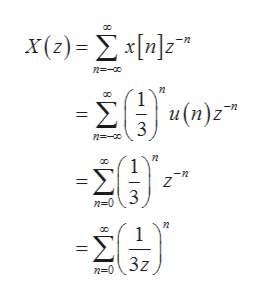# What will be the region of convergence of the Z transform of the discrete time sequence x(n)=(1/3)n u(n)?

Question
10 views

What will be the region of convergence of the Z transform of the discrete time sequence x(n)=(1/3)n u(n)?

check_circle

Step 1

Region of convergence: It is defined as the region in complex plane where z-transform of any discrete signal exists.

Step 2

Write the given discrete signal.

Step 3

Write the expression f...help_outlineImage TranscriptioncloseX(2) -Σ [n]z" η-- 2θo α(1)2 Σμ и (п)z 3 η-- θ η -η 3 η-0 3z n=0 || fullscreen

### Want to see the full answer?

See Solution

#### Want to see this answer and more?

Solutions are written by subject experts who are available 24/7. Questions are typically answered within 1 hour.*

See Solution
*Response times may vary by subject and question.
Tagged in

### Electrical Engineering